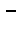Next: Finding Trigonometric Identities Up: The Problem Spaces Previous: The Problem Spaces

## Symbolic Regression

In the first problem we apply our system to a symbolic regression problem summarised in Table 1 [Ryan 98a]. The system is given a set of input and output pairs, and must determine the function that maps one onto the other. The particular function examined is X4 + X3 + X2 + X with the input values in the range [-1..+1]. As the target function has no constant values, only the variable X, it was decided to use only one terminal operand, i.e. X. So as not to unduly bias the system towards the correct solution, the terminal operators set consisted of more than the binary addition and multiplication operators, which alone would be sufficient to reach the target function. To determine the fitness of an individual program 20 test points were applied in the range -1 to 1, and the fitness was taken as the sum of the error.

Table 1: Grammatical Evolution Tableau for Symbolic Regression
 Objective : Find a function of one independent variable and one dependent variable,in symbolic form that fits a given sample of 20 (xi, yi) data points, where the target functions is the quartic polynomial X4 + X3 + X2 + X Terminal Operands: X (the independent variable) Terminal Operators The binary operators +, *,and, the unary operators Sin, Cos, Exp and Log Fitness cases The given sample of 20 data points in the interval [-1, +1] Raw Fitness The sum, taken over the 20 fitness cases, of the error Standardised Fitness Same as raw fitness Hits The number of fitness cases for which the error is less than 0.01 Wrapper Standard productions to generate C functions Parameters M = 500, G = 51Next: Finding Trigonometric Identities Up: The Problem Spaces Previous: The Problem Spaces
root
1998-10-02